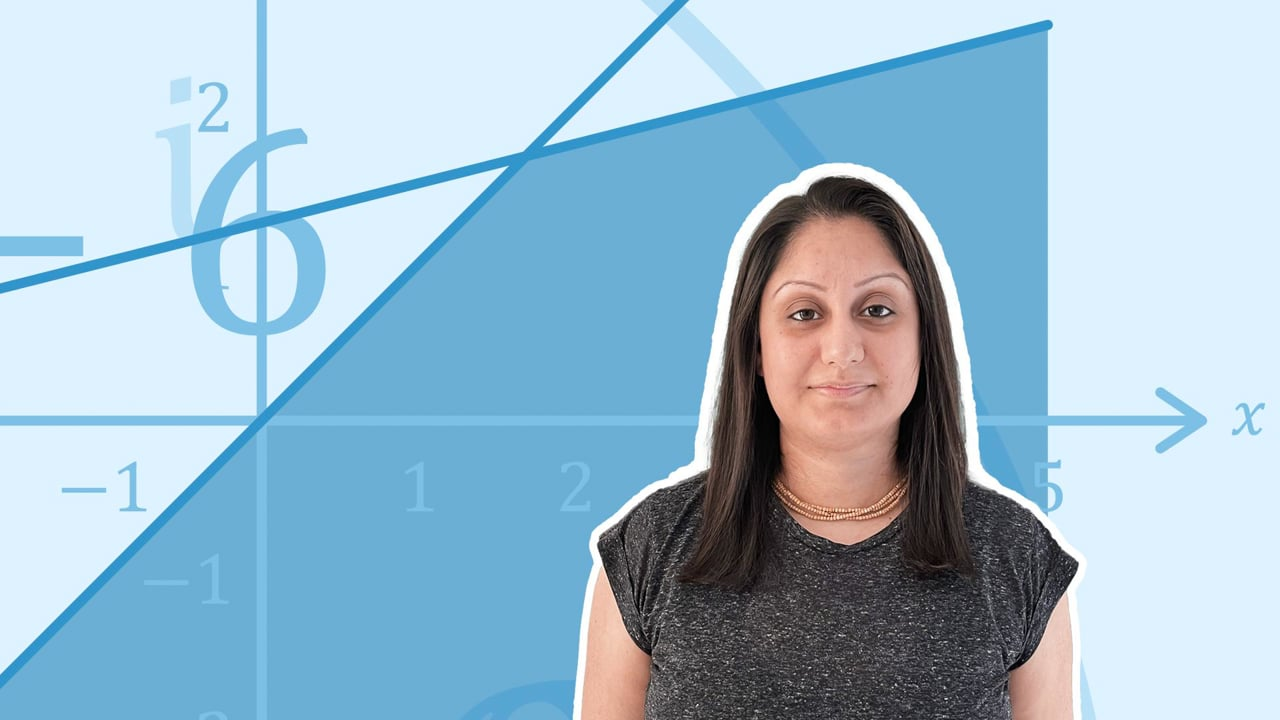Chapter overviewMaths

Types of numbers

Number calculations

Fractions, decimals and percentages

Algebraic manipulation

Formulae and equations

Straight line graphs

Other graphs

Ratio

Proportion

Rates of change

Shapes

Properties of shapes

Measures

Lines and angles

Drawing shapes

Trigonometry

Probability

Statistics

Maths

# The nth term0%

Summary

# The nth term

## In a nutshell

Sequences defined using an $n^{th}$ term formula help us find the sequence from the position of the terms in the sequence. The $n^{th}$ term method is more useful, as if we want to find the 100th term, it is not necessary to find any of the previous terms.

## Generating a sequence

The ​$n^{th}$ term formula can be used to generate a sequence. Start by substituting $n=1$ into the formula to find the first term. Then substitute $n=2$ for the second term, $n=3$ for the third term and so on.​

##### Examples

NTH TERM FORMULA

SEQUENCE

$n^{th} term = 4n+4$​​
$8,12, 16, 20,24$​​
$n^{th} term = 8-6n$​​
$2, -4, -10, -16, -22$​​
$n^{th} term = n^2+2$​​
$3, 6, 11, 18, 27$​​

## Find the nth term formula for an arithmetic sequence

An arithmetic sequence is one where the differences between adjacent terms are always the same. The term-to-term rule would be to $+$ or $-$ the same number each time.

It is possible to find the $n^{th}$ term formula for an arithmetic sequence.

##### Example

Find ​the $n^{th}$ term formula for the sequence $2, 5, 8, 11$.

Start by putting the sequence, and their positions $n$ into a table. Work out the difference each time, in this case the difference is $3$, so add another row in the table for $3n$. Then think about how to get from $3n$ to the sequence, in this case each term in our sequence is $1$ less than the $3n$ row, so the formula is $\underline{n^{th} \space term = 3n-1}$.

 ​$n$ (the position of each term) ​$1$​​ ​$2$​​ ​$3$​​ ​$4$​​ ​$n^{th} \space term$ (the sequence) ​$2$​​ ​$5$​​ ​$8$​​ ​$11$​​ the difference is $3$​ so use $3n$​​ ​$3$​​ ​$6$​​ ​$9$​​ ​$12$​​ each term is $1$ less than $3n$, so use $3n-1$​​ ​$2$​​ ​$5$​​ ​$8$​​ ​$11$​​

$\underline {n^{th} \space term = 3n-1}$

Note: Always check the formula, by substituting values for $n$ to see if it works.

FAQs

• Question: How do you find the nth term formula for an arithmetic sequence?

Answer: Start by finding the difference between term, this is the number that goes with n. For example, if the difference is +3, then use 3n. Then either add or subtract a constant until it matches the sequence.

• Question: Is nth term better than term-to-term rule?

Answer: The nth term method of defining a sequence can be more useful than the term-to-term rule, as it does not rely on finding any other previous terms in the sequence.

• Question: What is an nth term formula?

Answer: An nth term formula is a way of defining a sequence. The 'n' stands for the position of the term in the sequence. We can substitute different values of n to find the term.

Theory

Exercises